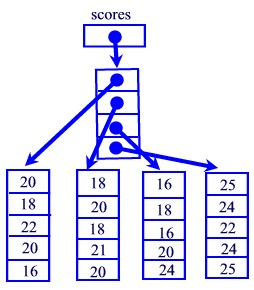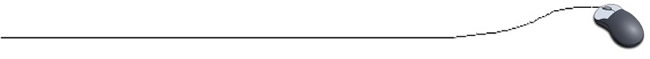Matrices - Two-dimensional arrays

Up until now, all of our arrays have been one-dimensional arrays.  These arrays have had "length", but the "width" (or height) remained as only one cell.We are now ready to discuss two-dimensional arrays, called matrices (singular:  matrix).  A matrix resembles a table with rows and columns.

It is possible for arrays to have multiple dimensions.  A three dimensional array, for example, has 3 subscripts, where each dimension is represented as a subscript in the array.  While it is possible for arrays to have any number of dimensions, most arrays are of one or two dimensions.

The elements of a matrix must be of the same data type.

Example:  The Computer Club is participating in a series of Programming Meets.  The table below shows the Club's results, where the maximum score for each contestant in a meet is 25.

 Name Meet  #1 Score Meet  #2 Score Meet #3 Score Meet #4 Score Meet #5 Score 1.  Robbins 20 18 22 20 16 2.  Montgomery 18 20 18 21 20 3.  Stevenson 16 18 16 20 24 4.  Norton 25 24 22 24 25
 Note:  Although the students' names and the meet numbers are shown, they are not part of the actual data and  are not part of the matrix.  Remember, the data in a two-dimensional array is always of the same data type. The data displayed in this table consists of 20 values -- four rows by five columns.  This matrix will have 20 cells, or elements. When you define storage for a matrix (a multi-dimensional array), you must specify that the array has more than one dimension by putting more than one subscript in brackets after the type designation.  The following declaration would be used to store the data shown in the table above: int [ ] [ ] scores = new int [ 4 ] [ 5 ] ;    // Declares a 2-D array This declaration creates a matrix with the following subscripted elements:
 columns rows                    

The code int  indicates that there will be four arrays of ints in the array scores, with 5 ints in each array of ints.

While we visualize this arrangement by thinking of a matrix of rows and columns, something slightly different is occurring in the memory.scores holds a reference to an array of 4 elements where each element is a reference to an array of 5 integers. It is easier to simply think of it as a grid or matrix.

At times, you may need to remember that each row is actually an array.  These "row" arrays can be referred to as scores, scores, scores, and scores where each row is type int [ ].

Working with Matrices:
• Filling by list -Just as working with a one-dimensional array, it is possible to fill a two dimensional array by using a list at the time the array is declared.  Notice the "sets" of braces "within" the list denoting the "array of arrays".

 int [ ] [ ] scores = {  }; { 20, 18, 22, 20, 16 }, { 18, 20, 18, 21, 20 }, { 16, 18, 16, 20, 24 }, { 25, 24, 22, 24, 25 } Notice the punctuation in the list.  The "arrays" are separated by commas, as are ordinary elements in a list.  Be sure you include the starting and ending (all inclusive) braces.

• Default filling when declared - When an array is created it is automatically filled with a zero (for numerical values), a false (for boolean values) or null (for String values).

• Filling with user input- When working with two-dimensional arrays (such as accessing, filling, printing, etc.), it is necessary to use nested loops.  The outer loop controls the number of rows and the inner loop controls the number of columns.

// Filling the matrix
import java.util.Scanner;
. . .

double [ ][ ] scores = new double   ;
for ( row = 0; row < 4; row ++ )
{
for ( column = 0; column < 5; column + + )
{
System.out.println("Enter score " + column + " for contestant " + row +":'");
scores [ row ] [ column ] =  reply.nextDouble();
}
}

• Manipulating a matrix - Suppose you want to save the information for 30 students and 3 exam grades for each student entered at the keyboard.  In addition, you want to find the average (which could be a decimal value)  for each student, and then store this average in a fourth column of the same matrix.  Remember, you will need to obtain the grades before you can compute the average.  Here is one possibility:

import java.util.Scanner;

public class matrixtest
{
public static void main(String[] args)
{
//create memory space for entire matrix
double [ ] [ ] grades = new double [ 30 ] [ 4 ] ;

// Fill the matrix with user input and compute average
int row, column;
double sum, average, temp;
for ( row = 0; row < 3; row ++ )
{
sum = 0;
for(column = 0; column < 3; column++)
{
System.out.println("Enter grade "+(column+1)+" for contestant "+(row+1)+":'");

}
average = sum / 3;
}

// Print averages only
System.out.println("You saved the following averages: ");
for( row = 0; row < 3; row ++ )
{
System.out.println("Student " + (row + 1) + ": " + grades[row]);
}
}
}

Length:  Just as a command such as list.length returns the length of a one dimensional array, scores.length will return the number of rows in this two-dimensional array.   scores[ i ].length will return the number of columns of the row with subscript i in a two-dimensional array.

• Working with Strings - Create a matrix of String values, fill the matrix by list, and print the matrix.  Notice that the "internal" arrays are of differing sizes.  Notice how the .length is used to deal with these varying lengths during printing.

public class ArrayOfArraysAnimalDemo
{
public static void main(String[ ] args)
{
String[ ][ ] animals = {
{ "DanaDog", "WallyDog", "JessieDog", "AlexisDog", "LuckyDog" },
{ "BibsCat", "DoodleCat", "MillieCat", "SimonCat" },
{ "ElyFish", "CloieFish", "GoldieFish", "OscarFish", "ZippyFish", "TedFish"},
{"RascalMule","GeorgeMule","GracieMule","MontyMule","BuckMule","RosieMule"}
};
// printing matrix
for (int i = 0; i < animals.length; i++)
{
System.out.print(animals[ i ] [ 0 ] + " ");
for (int j = 1; j < animals[ i ].length; j++)
{
System.out.print(animals[ i ][ j ] + " ");
}
System.out.println( );
}
}
}